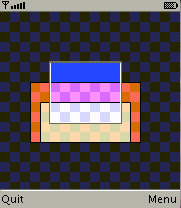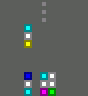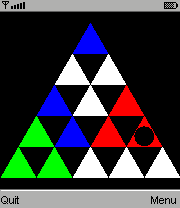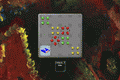-=+=- -=+=- -=+=- -=+=- -=+=- -=+=- -=+=- -=+=- -=+=- -=+=- -=+=- -=+=- -=+=- -=+=- -=+=- -=+=- -=+=- -=+=- -=+=- -=+=- -=+=- -=+=- -=+=- -=+=- -=+=- -=+=- -=+=- -=+=- -=+=- -=+=- (c) WidthPadding Industries 1987 0|712|0 -=+=- -=+=- -=+=- -=+=- -=+=- -=+=- -=+=- -=+=- -=+=- -=+=- -=+=- -=+=- -=+=- -=+=- -=+=- -=+=- -=+=- -=+=- -=+=- -=+=- -=+=- -=+=- -=+=- -=+=- -=+=- -=+=- -=+=- -=+=- -=+=- -=+=-

Pio# Exploding Numbers

29th January 2023Divide and add numbers in 2D grid to reach a stated goal

Pio# Twinnings

20th November 2022Find pairs

Pio# POCA (reboot)

26th December 2020Remove all arrows without geting hit

Pio# SlotM

27th July 2018A simple simulation of slot machine.

Pio13th April 2015Game and watch

Pio# Jump

2nd March 2010One button rope skipping

Pio# Entangled rectangles mobile

12th April 2009Overlap the rectangles

Pio# Generic Block Game

16th September 2008Configurable falling blocks game

Pio# Ruben's Pyramid

20th March 2008actually a triangle; shuffle then solve

Pio# Arecibo

16th February 2008

tile drawing web page

Pio# Volcano game (untitled)

16th January 2008remember where you clicked and imagine the position of tiles (update:01-26-08)

Pio# Poca

11th January 2008remove all arrows

More - Older Posts